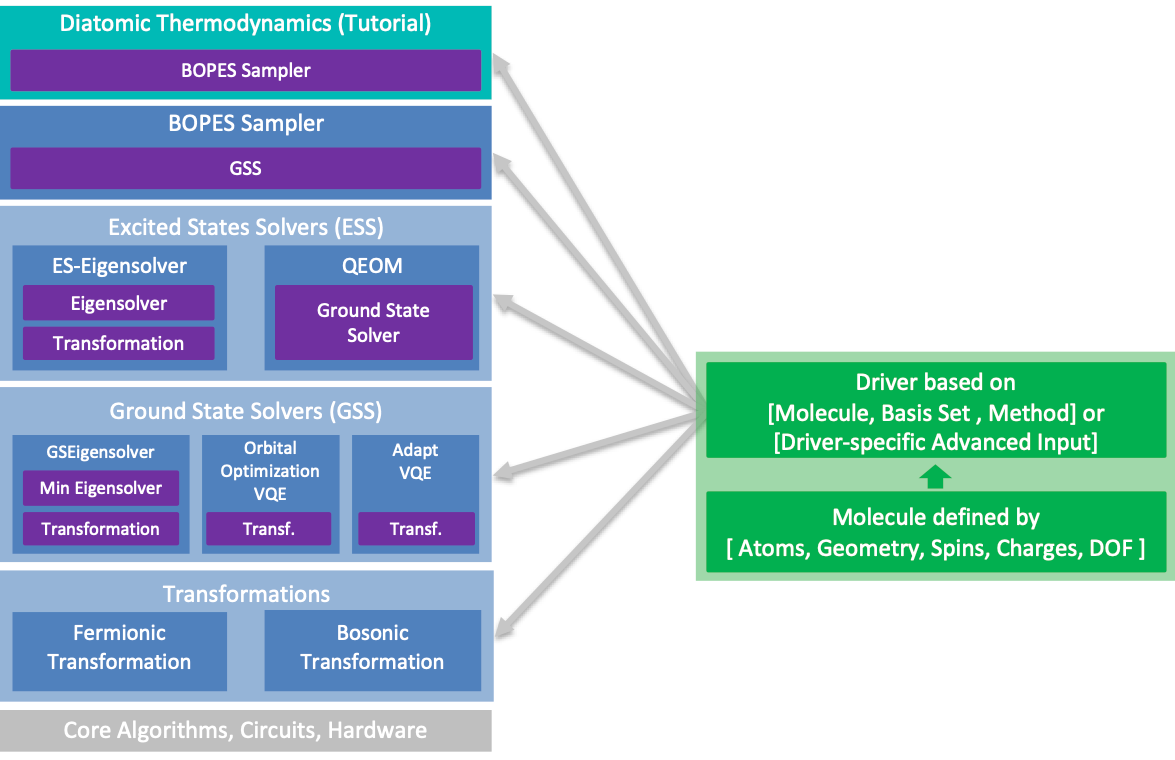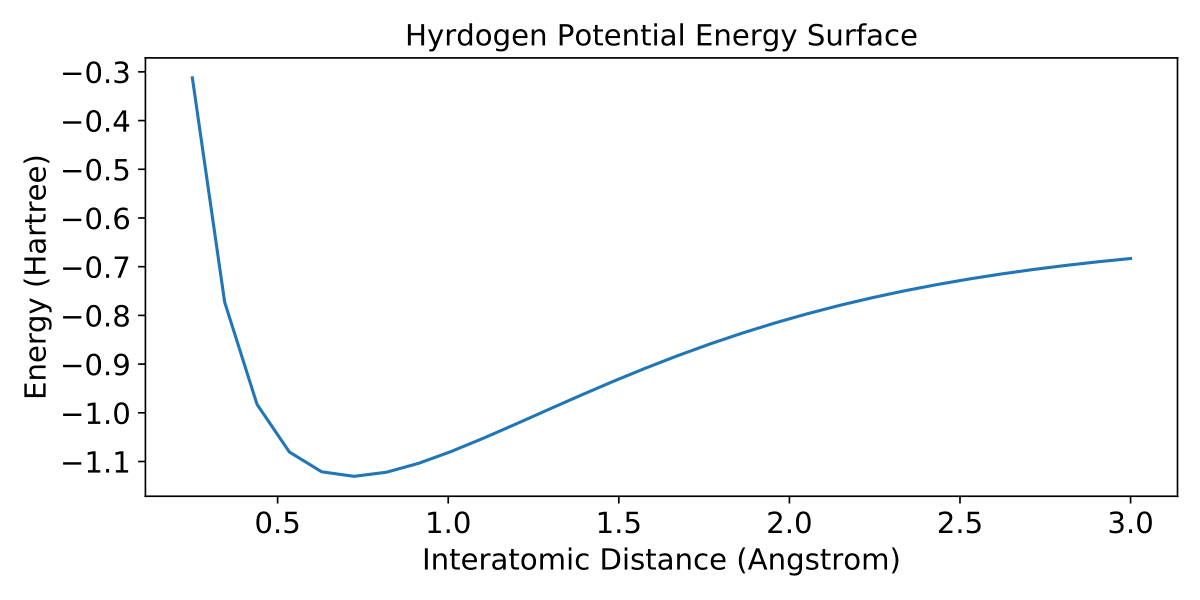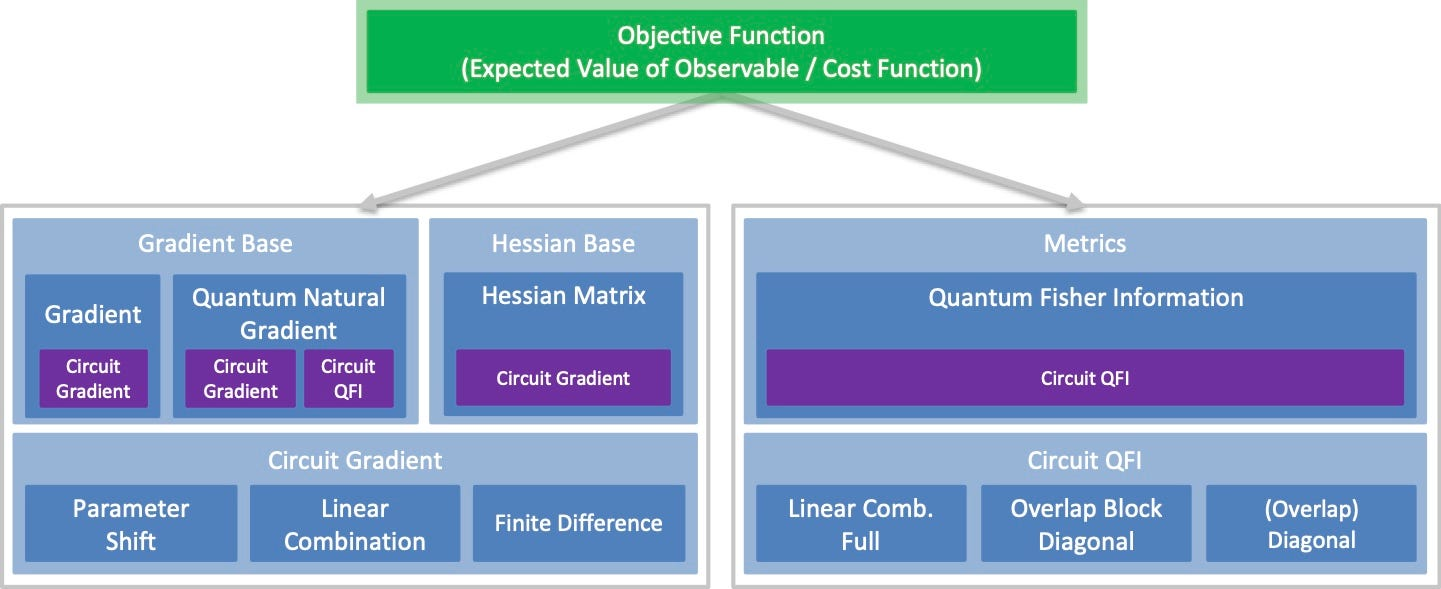News

# Introducing The New Qiskit Chemistry Module And Gradients Framework For Next-Level Quantum ComputingToday, we’re excited to announce a complete overhaul of the Qiskit Chemistry module, plus the new Qiskit Gradients framework, meant both for quantum application developers as well as domain experts with basic knowledge of quantum computing. These enhancements represent an important step toward frictionless quantum computing, where developers can write applications reaping the benefits of quantum computers without concerning themselves with the intricacies of the hardware.

The team designed the new Qiskit Chemistry module to be both modular and extensible, while providing high-level applications that make programming more intuitive for anyone interested in quantum computing. The module includes algorithms for calculating molecules’ electronic and vibronic structure, plus algorithmic primitives to serve as the building blocks for higher level applications. Recently, we teamed up with ExxonMobil scientists to compute thermodynamic observables for the hydrogen molecule on the ibmq_valencia quantum processor using Qiskit.

“The development of the new Qiskit Chemistry module is both exciting and very important. It will enable scientists with limited exposure to quantum hardware to quickly begin to simulate interesting and relevant chemistry problems. Perhaps as important, this new module will also help scientists think ‘in a quantum way’ when solving some of our most challenging problems,” said Laurent White, Section Head, Computational Physics, ExxonMobil Research and Engineering

Many near-term quantum algorithms — including the ones available in the Qiskit Chemistry module — are variational, i.e., they use classical optimization to find a set of parameters which minimize some target function evaluated using a quantum computer. These kinds of algorithms rely on efficient and robust optimization to serve purposes in combinatorial problems or quantum machine learning — and in the quantum chemistry case, the objective is an energy.

Meanwhile, the new Qiskit Gradients framework provides an automated way to compute analytic gradients — basically, how the circuit is changing as the variational algorithm runs — as well as functions of the gradients for a variety of problem classes. This is achieved by automatically constructing the operators required to estimate circuit derivatives and combining this with classical automatic differentiation. The Gradients framework not only supports the estimation of first order gradients but also Hessian and Quantum Fisher Information matrices. This immediately paves the way to more advanced algorithms like Quantum Natural GradientsVariational Quantum Imaginary / Real Time Evolution, and Variational Gibbs State Preparation. The Gradients framework is integrated into Qiskit’s core algorithms, making it straightforward to leverage in existing applications. Further, it will serve as a building block for future application modules, such as quantum machine learning.

## The Qiskit Chemistry Module

Simulating quantum systems on classical computers has been one of the greatest challenges for the quantum physics and quantum chemistry communities for the past few decades. Classically, we must use approximations to simulate larger systems due to the inherent complexity, but quantum computers can capture the intricate details of quantum systems like molecules, making these devices appealing for improved accuracy as well as speed. Quantum computing offers the possibility to efficiently simulate problems like the electronic structure of molecular systems, with the potential to help us discover new chemical reactions or new materials. Here, we introduce the new Qiskit Chemistry module, which is outlined in the following figure and which is a step toward harnessing the power of quantum computers for applications in quantum chemistry and physics.Figure 1: Qiskit Chemistry Module —Enables ground and excited state calculations. These algorithmic components can be reused throughout the stack. This equips users to easily build applications that leverage core algorithms, circuits, and quantum hardware. The transformations can transform a given driver into qubit operators and determine whether a problem is treated in a fermionic or bosonic context.

When solving quantum chemistry problems with a quantum computer, we often need to first calculate additional classical quantities. For instance, before we can use quantum techniques to compute any ground state property of a molecule, such as its energy or dipole moment, we need to compute one and two-electron integrals classically. This preprocessing step can be done efficiently using classical computers and is available through numerous classical software tools. To ensure that it works well with such tools, the Qiskit Chemistry module provides a consistent framework, called the Drivers, to define electronic structure or vibronic structure problems based on molecular properties such as geometry, charges and spins. In this way users can specify a chemical system, e.g. a molecule, and automatically calculate the classical properties required to generate the corresponding Fermionic or Bosonic Hamiltonian. To ensure that any problem’s Hamiltonian can be expressed as a Qubit Operator, this Qiskit release introduces Qubit Transformation interfaces for mapping fermionic and bosonic problems. For example, in the fermionic case the mapping from a Hamiltonian to a qubit operator can be done using different mappings such as the Bravyi-Kitaev, Jordan Wigner, or parity mapping. Once the problem is mapped into a form that we can evaluate on quantum hardware, the chemistry module can further simplify the operator with different symmetry reduction schemes. By providing control over each step in this problem translation procedure, the chemistry module empowers users to squeeze the maximum performance out of techniques designed for near-term quantum devices.

## Ground State Solver

A fundamental algorithmic building block of the Chemistry module is the Ground State Solver, i.e., the ability to approximate the ground state for a given problem. We provide multiple implementations such as the Groundstate Eigensolver, which first handles the translation from the problem instance (given as a Driver) to a Qubit Hamiltonian, then calls a Minimum Eigensolver such as the Variational Quantum Eigensolver (VQE), and finally translates the results back to an electronic or vibronic structure result depending on the provided transformation. Qiskit Chemistry offers different predefined variational circuits, such as the Unitary Coupled Cluster with Single and Double Excitations (UCCSD) ansatz, but the algorithms are also compatible with general parametrized quantum circuits and can leverage Qiskit’s Circuit Library. Further, the release includes some more targeted algorithms such as AdaptVQE and Orbital Optimization VQE that can be used for electronic structure calculations and exploit further information on the problem to approximate ground states more efficiently.

## Excited States Solver

In addition to approximating ground states, it is also important to be able to compute excited states and their corresponding energies. To this extent, Qiskit Chemistry offers Excited States Solvers such as a generic Eigensolver-based calculation that can be used with generic eigensolvers as well as the Quantum Equation of Motion (QEOM) algorithm. QEOM takes a Ground State Solver and leverages its results together with auxiliary excitation operators in order to evaluate the relevant commutators and compute the excited-state energies. Again, based on the provided transformation, Excited States Solvers can be used for electronic structure as well as vibronic structure calculations alike.

## BOPES Sampler

The Ground State and Excited States Solvers allow end users to evaluate properties of a given molecule. However, for many applications it is also important to see how properties change e.g. with changing molecular geometry or by varying other input parameters. With this release, Qiskit Chemistry supports a generic Molecule object that allows perturbation of different degrees of freedom (e.g. interatomic distance, molecule bending etc.). This is leveraged in the Born-Oppenheimer Potential Energy Surface (BOPES) Sampler to screen a range of different geometries and approximate the BOPES. To speed the computation up, the sampler also supports bootstrapping or extrapolating using previous calculations. The BOPES Sampler can take any Ground State Solver provided by the user to estimate the BOPES. Having access to the BOPES enables further applications such as fitting analytic functions to the potential energy surface and eventually the calculation of vibronic energies and partition functions. This has been demonstrated in the previously mentioned work by ExxonMobil and IBM Quantum, and we also provide a tutorial showcasing it. The following code snippet outlines how to set up the BOPES Sampler using VQE and produce the dissociation profile of a Hydrogen molecule.Figure 2: The Hydrogen potential energy surface computed by the BOPES Sampler.

Most near-term quantum algorithms include optimizing classical parameters, e.g., to minimize energies, costs, or loss functions. Thus, it is crucial to make this optimization as efficient and powerful as possible. To this end we introduce the Qiskit Gradients framework. This combines classical and quantum automatic differentiation techniques to automatically construct the quantum circuits and classical logic needed to estimate analytic gradients. An overview of the new components introduced in the Qiskit Gradients framework is given in Figure 4.Figure 3: Qiskit Gradients Framework — Supports first and second order derivatives based on three different approaches to construct circuit gradients. Furthermore, the Quantum Fisher Information matrix can be evaluated which allows users to automatically evaluate the Quantum Natural Gradient and enables other sophisticated algorithms.

For some variational optimization applications, we are interested in tuning not only parameterized quantum circuits, but also parameterized Hamiltonians. This can be useful when optimizing mixed integer programs as well as searching over molecular configurations. Of course, the Gradients framework is also applicable to these settings by combining classical automatic differentiation with automatic generation of circuit gradients and is, thus, able to evaluate the problem derivatives.

Multiple approaches to evaluate circuit gradients are supported by Qiskit’s Gradients framework: parameter shift, linear combination of unitaries, and finite difference. Parameter shift gradients allow parametrized circuits to be reused as given and only require some adjusting of parameters. However, because parameter-shift gradients require two circuit evaluations per derivative, the variance of the estimator is increased. By contrast, the linear combination approach achieves a lower variance, but requires the introduction of an additional working qubit and intercepting controlled gates. Thus, both types have pros and cons and need to be selected based on the context. Lastly, finite difference is a straight-forward numerical approach which is generally applicable, but in contrast to the first two methods it only approximates the gradient and thus may lead to worse performance.

## Supported Problem Types

In many near-term quantum algorithms, a common subroutine involves minimizing the expected value of some quantum mechanical observable. These observables often correspond to energies, costs, or loss functions and they arise in diverse contexts such as quantum chemistry, physics, combinatorial optimization, and machine learning. Thus, the evaluation of gradients when estimating observables is a well-motivated primitive now directly supported by Qiskit. However, for more complex loss functions, one may require the evaluation of probability gradients, i.e., the gradient of the sampling probabilities for a particular measurement basis. Fortunately, the evaluation of probability gradients is also supported and can be used to evaluate the gradients of a more general class of loss functions such as the log-likelihood. The chemistry code snippet shown above leverages the Gradient within VQE and illustrates how seamlessly it can be integrated into existing workflows.

## Higher-Order Derivatives and Metrics

Qiskit’s Gradients framework also supports the evaluation of the Hessian matrix, i.e., of pairwise second order derivatives. The Hessian can be used to define higher-order optimization schemes; however, this comes at the cost of requiring more circuits to be computed per iteration. The impact of using the Hessian matrix, particularly in the presence of noise is an open research question which requires further investigation.

In addition to first and second order derivatives, the gradients framework also enables automatic computation of the Quantum Fisher Information (QFI) matrix. The QFI is a metric tensor which can give us insight into how well a quantum circuit can represent functions. It is also of particular interest as it can help to improve the optimization landscape by mapping the gradient into a feature space that takes into account the information geometry of the problem, i.e., by using Quantum Natural Gradients. We allow three different evaluations of the QFI: First, the full QFI via a linear combination approach. Second, a block-diagonal QFI via the overlap approach, where the blocks are defined by one layer of parametrized gates in the variational circuit. And last, the diagonal of the QFI, again by the overlap approach. Although the linear combination of unitaries method is exact, the overlap approximations do not require the use of working qubits or intercepting controlled gates and may find use depending on the context. In addition to the Quantum Natural Gradient, Qiskit’s automated computation of QFI metric tensors enables sophisticated applications such as Variational Quantum Imaginary / Real Time EvolutionGibbs State Preparation, or Variational Quantum Boltzmann Machines.

## How to get started

The easiest way to explore the tools introduced above is to install the latest Qiskit version and start playing around with the newly released tutorial notebooks for chemistry and gradients. In addition, you can check out the Qiskit slack workspace to connect with other Qiskit users and contributors.